# Python Lists

Lists in python are also a type of sequences just like tuples.

Unlike tuples, lists are mutable, that is, lists can be modified. Therefore, lists have all the features that tuples have, plus another feature is, it can be modified.

A list basically contains items separated by commas and enclosed within the square brackets [].

Items in the list needn't be of the same type.

## Creating a List in Python

Here is an example shows how to create a list in python.

```list1 = ['computer', 'programming', 1957, 2070, 3242];
list2 = [1, 2, 3, 4, 5];
list3 = ["a", "b", "c", "d", "e"];```

Now let's take another example of creating a list in python.

```# Python Lists Example - Creating a list program
my_list = ["zero", "one", "two", "three"];
print("Elements of the list, my_list are:");
for ml in my_list:
print(ml);```

Here is the screenshot of the sample output that you will see on your output screen after executing the above example code of creating a list in python.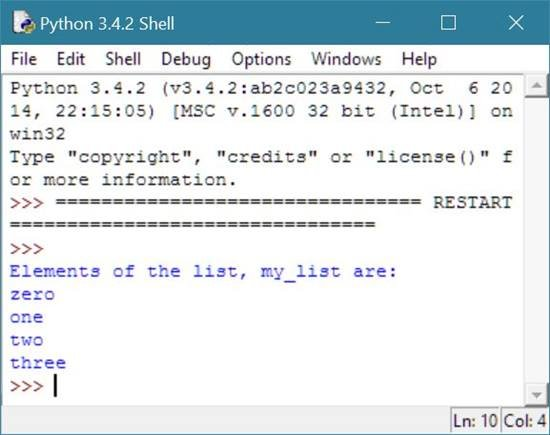## Python Lists Example

Here is an example program, demonstrating the concept and use of list in python:

```# Python Lists - Example Program

list1 = ["python", "list", 1952, 2323, 432]
list2 = ["this", "is", "another", "list"]

print(list1)              # this will print the complete list
print(list1[1:4])         # this will print the elements starting from 2nd till 4th
print(list1[1:])          # this will print the elements starting from the 2nd element
print(list1)           # this wil print the first element of the list
print(list1 * 2)          # this will print the list two times
print(list1 + list2)      # this will print the concatenated list```

Here is the sample output of the above python program: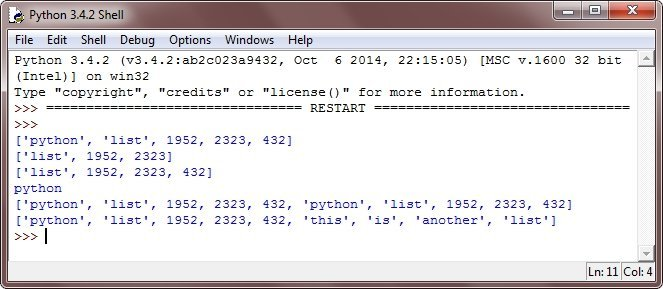## Find Length of List in Python

To find the length of a list in python, use len() function as shown in the example given below.

```# Example of finding length of list in python
my_list = ["one", "two", "three", "four"];
length_of_list = len(my_list);
print("Length of the list, my_list is:",length_of_list);```

If you will execute the above example of printing or finding the length of the list in python, then you will see the following output: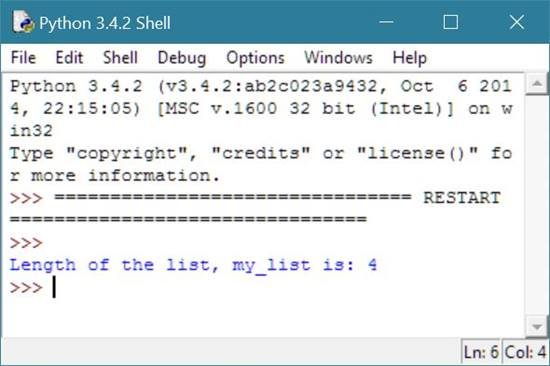## Check Element is in List or Not

To check whether a particular element or item is present inside the list or not in python, then use in operator as shown in the example here.

```# Check an element is in the list or not in python example
my_list = ["one", "two", "three", "four"];
if "two" in my_list:
print("'two' is in the list, my_list.");
else:
print("'two' is not in the list, my_list.");```

Below is the sample output produced by the above example of checking an element is in the list or not in python.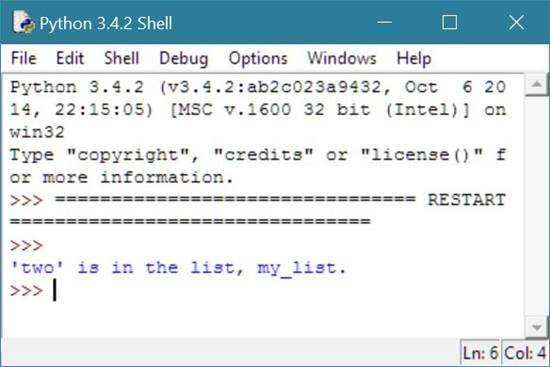Now let's modify the above code with below one.

```my_list = ["one", "two", "three", "four"];
element = input("Enter element:");
if element in my_list:
print(element,"is in the list, my_list.");
else:
print(element,"is not in the list, my_list.");```

Here is the initial output produced by the above example of checking item is in the list or not in python.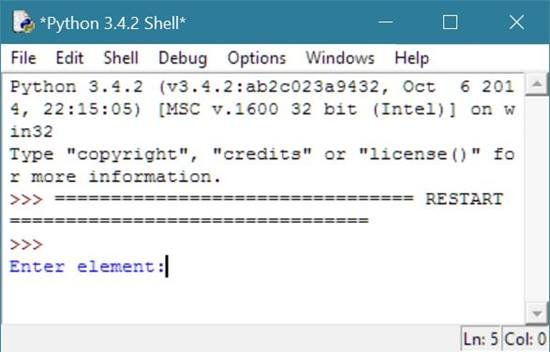Now enter or type element, say one and press enter to see the following output.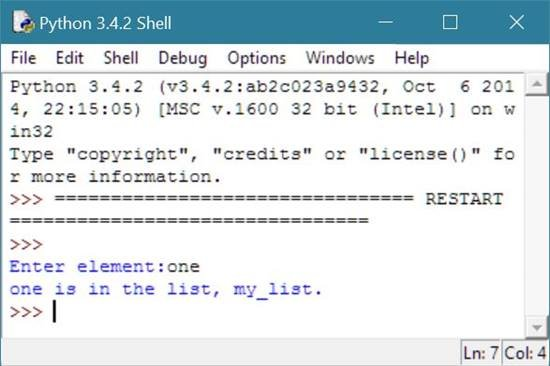and type element, say seven and press enter to see the following output this time:## Indexing Lists in Python

Indexing lists in python, means finding the element or item present inside the list by its index number as shown in the example given below.

Note - Index starts from 0.

```# Indexing a List in Python Example
my_list = ["one", "two", "three", "four"];
index_no = input("Enter index number:");
index_no = int(index_no);
print(my_list[index_no],"is present at this index number.");```

If you will execute the above example of indexing a list in python, then here is the sample and initial output produced.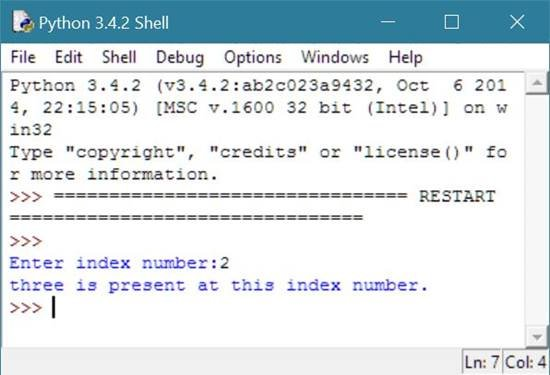## Slicing Lists in Python

Slicing lists in python can be done in same way as done in tuple case. That is, you have to provide beginning and ending index number to slice all the element or item present in between these two index numbers from the list in python as shown in the example given below.

```# Slicing Lists in Python Example
my_list = ["one", "two", "three", "four", "five"];
start_index_no = input("Enter starting index number:");
end_index_no = input("Enter ending index number:");
start_index_no = int(start_index_no);
end_index_no = int(end_index_no);
print("\nElements present in between these two index numbers are:");
print(my_list[start_index_no:end_index_no]);```

Here is the initial output produced after executing the above example of slicing lists in python.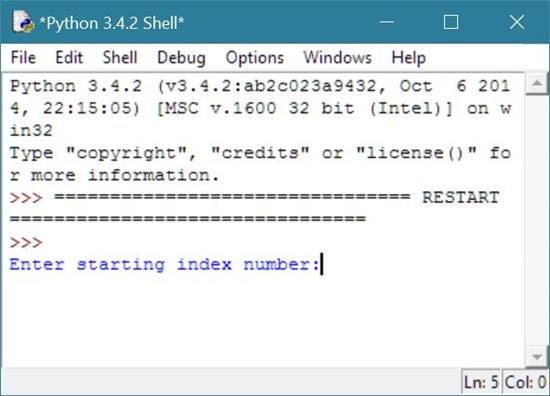Now provide beginning and ending index number, say 2 and 5 and press enter key to see the following output: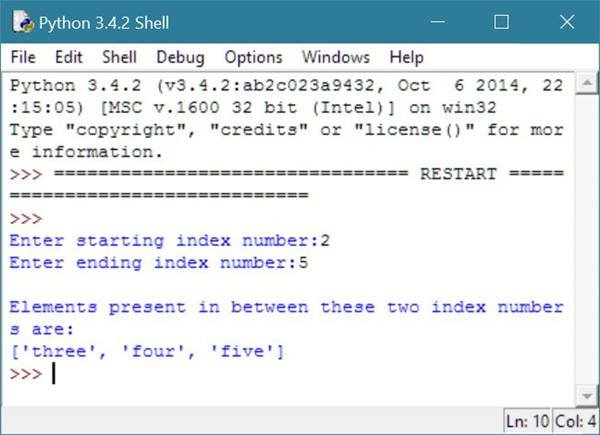## Concatenating Lists in Python

Concatenating two lists in python means joining them together and form one lists that contains all the elements of both the list as shown in the example given below.

```# Lists concatenation in python example
my_list = ["zero", "one", "two", "three", "four"];
my_new_list = ["five", "six"];
my_list += my_new_list;
print("List's items after concatenating:");
for l in my_list:
print(l);```

Here is the sample output after executing the above example of concatenating lists in python.Now let's modify the above example of concatenating lists with below one in python.

```my_list = ["zero", "one", "two", "three", "four"];
my_new_list = ["five", "six"];
concatenated_list = my_list + my_new_list;
print("Elements of list, my_list are:");
for ml in my_list:
print(ml);
print("\nElements of list, my_new_list are:");
for mnl in my_new_list:
print(mnl);
print("\nElements of list, concatenated_list are:");
for cl in concatenated_list:
print(cl);```

Following is the sample output of above example.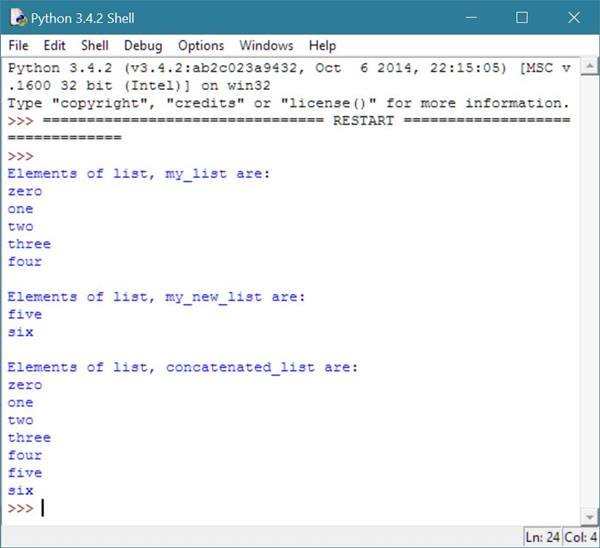## Replacing List Element with new one in Python

Since lists in python is mutable, that is, you can modify the list unlike tuple. Therefore here is an example shows how to replace old element of the list with new element in python.

```my_list = ["zero", "one", "two", "three", "four"];
print("Elements of the list, my_list are:");
for ml in my_list:
print(ml);
my_list = "one";
my_list = "five";
print("\nNow elements of the list, my_list are:");
for ml in my_list:
print(ml);```

Below is the sample output of the above example of lists in python to replace list's element with new one.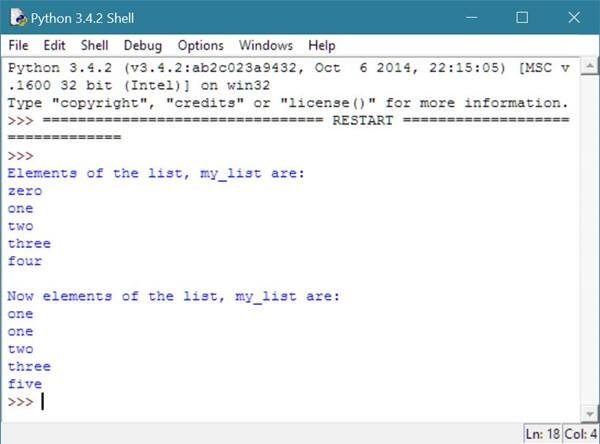## Python Assigning New Element in List

Here is an example shows how to assign new list element in python using list slice.

```my_list = ["zero", "one", "two", "three", "four"];
print("Elements of the list, my_list are:");
for ml in my_list:
print(ml);
my_list[5:5] = ["five"];
my_list[6:6] = ["six"];
print("\nNow elements of the list, my_list are:");
for ml in my_list:
print(ml);
print("\nElement present at index number 6 is:",my_list);```

Below is the sample output of above assigning new list element in python example code.## Deleting Element from List in Python

To delete any element from a list in python, use del keyword followed by the list name with its index number inside square bracket, that is, for example, say my_list[index] or my_list as shown in the example given below.

```# Deleting element from list in python example
my_list = ["zero", "one", "two", "three", "four"];
print("Elements of the list, my_list are:");
for ml in my_list:
print(ml);
index = input("\nEnter index no:");
index = int(index);
print("Deleting the element present at index number",index);
del my_list[index];
print("\nNow elements of the list, my_list are:");
for ml in my_list:
print(ml);```

Following is the initial output produced by the above example code of deleting list element in python.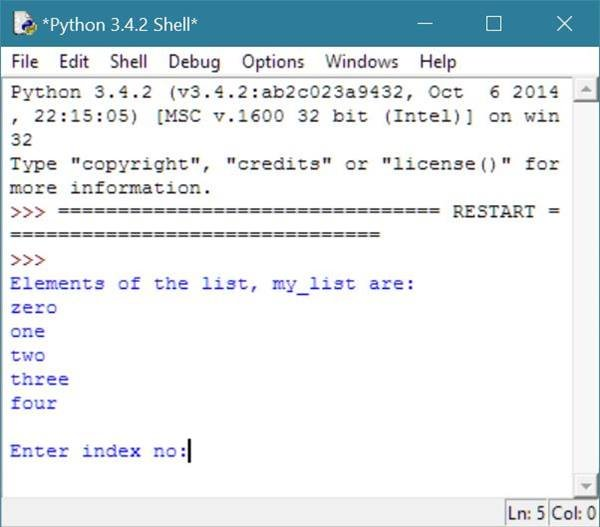Now enter the index number say 0 to delete the element present in the list at that index number, that is 0 as shown in the output given below.Here is another example, also shows how to delete element from the list, but using slice, that is, you are free to delete all the elements using slicing list technique. If you will do this, then all the element present in between will be deleted, and you don't have to provide one by one index number to delete all these elements as shown in the example given below.

```my_list = ["zero", "one", "two", "three", "four"];
print("Elements of the list, my_list are:");
for ml in my_list:
print(ml);
del my_list[2:4];
print("\nNow elements of the list, my_list are:");
for ml in my_list:
print(ml);```

Below is the sample output of the above deleting lists element in python example code.## Python Nested Lists

You can also use nested sequences in your python program using python lists as shown in the following example.

```# Nested lists in python example
books_list = [("C", 896), ("C++", 599), ("Python", 1269)];
print("Books list with prices.");
ch = None;
while ch != "0":
print("\n0 - Exit");
print("1 - Show Books List");
ch = input("Choice:");
if ch == "0":
print("Exiting...");
elif ch == "1":
print("\nBook\t\tPrice");
print(book_name,"\t\t",book_price);
elif ch == "2":
book_name = input("\nEnter name of the book:");
book_price = int(input("Enter its price:"));
else:
print("Sorry, but",choice,"is not valid choice.");```

In the above example of nested lists in python, append() method is used to append new elements in the list.

Below is the initial output produced by the above program of nested sequences using list in python.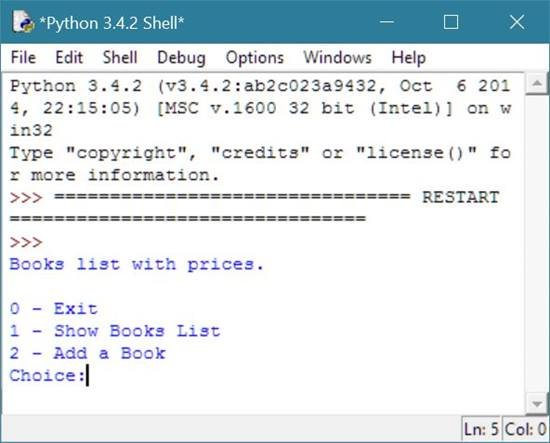Now, type or enter 1 as choice and press enter to see the following output.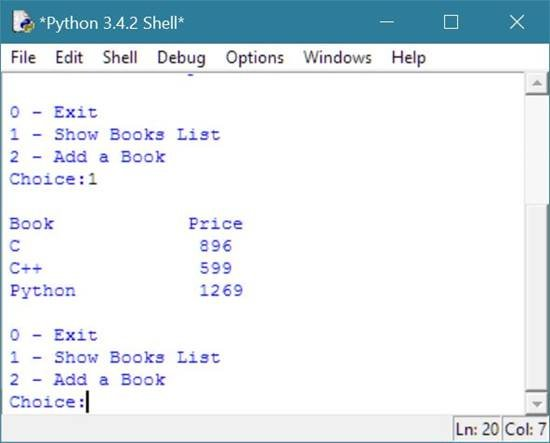Now enter 2 and provide the detail of new book to add another book in the list as shown in the following output.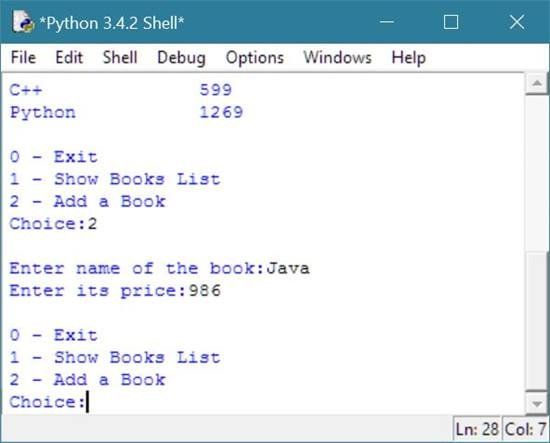Now, give 1 as choice and press enter to list down all the books with its prices to see the newly added book in the record or list.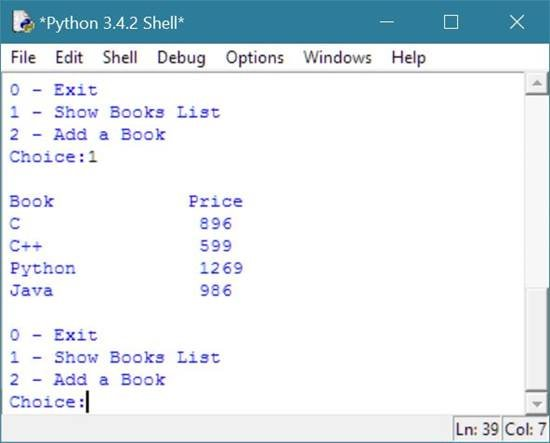Now, type 0 to exit as shown in the following screenshot. This is the fullscreen of the output window.Tools
Calculator